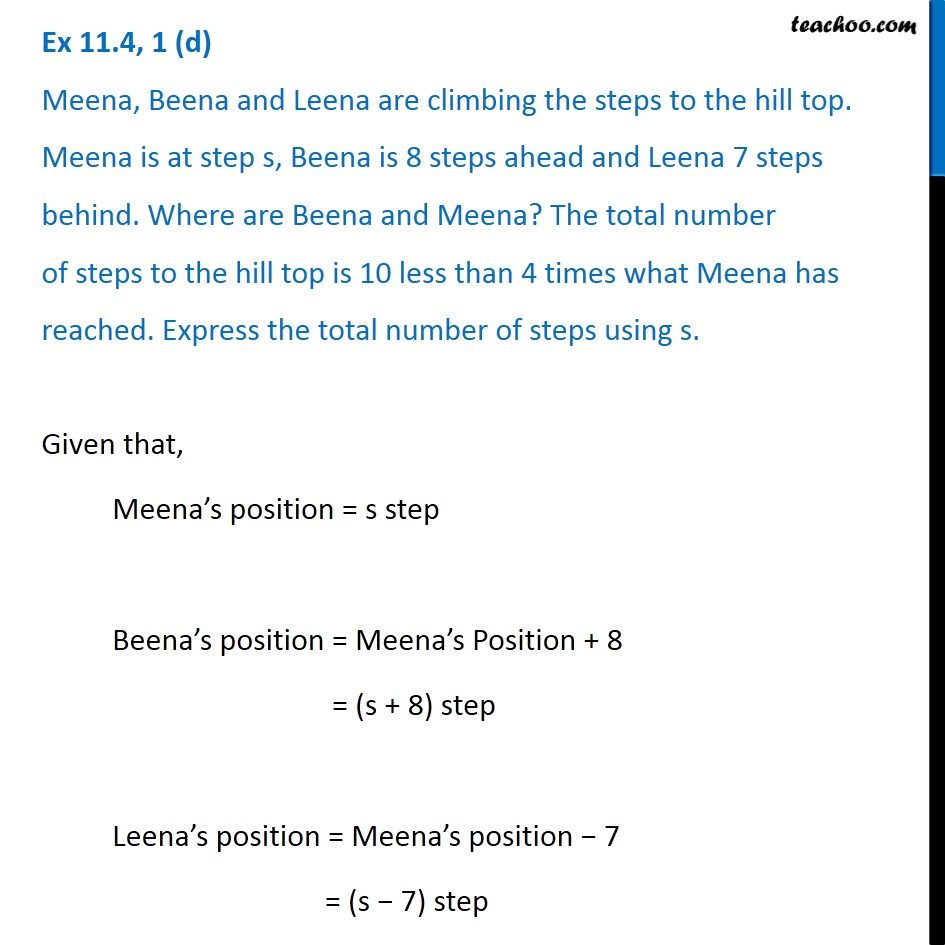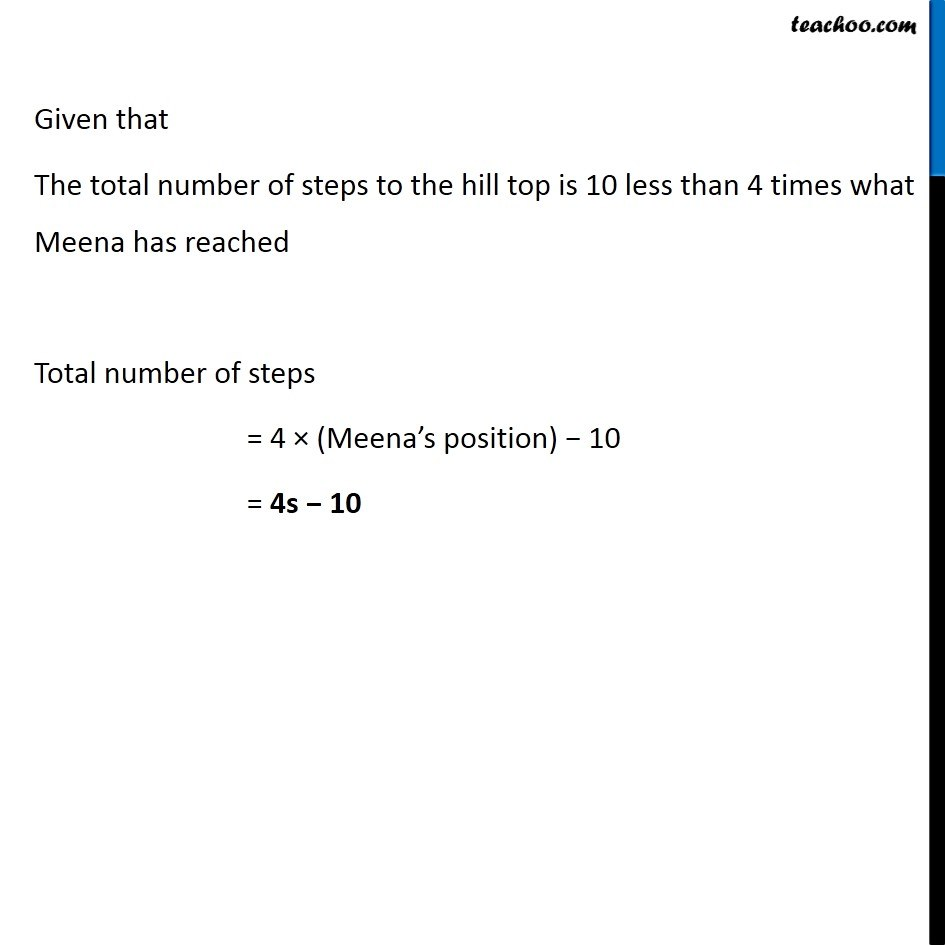1. Chapter 11 Class 6 Algebra
2. Concept wise
3. Statements to expressions

Transcript

Ex 11.4, 1 (d) Meena, Beena and Leena are climbing the steps to the hill top. Meena is at step s, Beena is 8 steps ahead and Leena 7 steps behind. Where are Beena and Meena? The total number of steps to the hill top is 10 less than 4 times what Meena has reached. Express the total number of steps using s. Given that, Meena’s position = s step Beena’s position = Meena’s Position + 8 = (s + 8) step Leena’s position = Meena’s position − 7 = (s − 7) step Given that The total number of steps to the hill top is 10 less than 4 times what Meena has reached Total number of steps = 4 × (Meena’s position) − 10 = 4s − 10